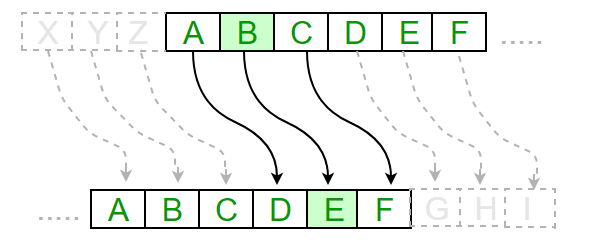# Caesar Cipher in Cryptography

• Difficulty Level : Easy
• Last Updated : 07 Jul, 2022

The Caesar Cipher technique is one of the earliest and simplest methods of encryption technique. It’s simply a type of substitution cipher, i.e., each letter of a given text is replaced by a letter with a fixed number of positions down the alphabet. For example with a shift of 1, A would be replaced by B, B would become C, and so on. The method is apparently named after Julius Caesar, who apparently used it to communicate with his officials.

Thus to cipher a given text we need an integer value, known as a shift which indicates the number of positions each letter of the text has been moved down.
The encryption can be represented using modular arithmetic by first transforming the letters into numbers, according to the scheme, A = 0, B = 1,…, Z = 25. Encryption of a letter by a shift n can be described mathematically as.(Encryption Phase with shift n)(Decryption Phase with shift n)Examples :

```Text : ABCDEFGHIJKLMNOPQRSTUVWXYZ
Shift: 23
Cipher: XYZABCDEFGHIJKLMNOPQRSTUVW

Text : ATTACKATONCE
Shift: 4
Cipher: EXXEGOEXSRGI```

Algorithm for Caesar Cipher:
Input:

1. A String of lower case letters, called Text.
2. An Integer between 0-25 denoting the required shift.

Procedure:

• Traverse the given text one character at a time .
• For each character, transform the given character as per the rule, depending on whether we’re encrypting or decrypting the text.
• Return the new string generated.

A program that receives a Text (string) and Shift value( integer) and returns the encrypted text.

## C++

 `// A C++ program to illustrate Caesar Cipher Technique``#include ``using` `namespace` `std;`` ` `// This function receives text and shift and``// returns the encrypted text``string encrypt(string text, ``int` `s)``{``    ``string result = ``""``;`` ` `    ``// traverse text``    ``for` `(``int` `i=0;i

## Java

 `//A Java Program to illustrate Caesar Cipher Technique``class` `CaesarCipher``{``    ``// Encrypts text using a shift od s``    ``public` `static` `StringBuffer encrypt(String text, ``int` `s)``    ``{``        ``StringBuffer result= ``new` `StringBuffer();`` ` `        ``for` `(``int` `i=``0``; i

## Python3

 `#A python program to illustrate Caesar Cipher Technique``def` `encrypt(text,s):``    ``result ``=` `""`` ` `    ``# traverse text``    ``for` `i ``in` `range``(``len``(text)):``        ``char ``=` `text[i]`` ` `        ``# Encrypt uppercase characters``        ``if` `(char.isupper()):``            ``result ``+``=` `chr``((``ord``(char) ``+` `s``-``65``) ``%` `26` `+` `65``)`` ` `        ``# Encrypt lowercase characters``        ``else``:``            ``result ``+``=` `chr``((``ord``(char) ``+` `s ``-` `97``) ``%` `26` `+` `97``)`` ` `    ``return` `result`` ` `#check the above function``text ``=` `"ATTACKATONCE"``s ``=` `4``print` `(``"Text  : "` `+` `text)``print` `(``"Shift : "` `+` `str``(s))``print` `(``"Cipher: "` `+` `encrypt(text,s))`

## C#

 `// A C# Program to illustrate Caesar Cipher Technique``using` `System;``using` `System.Text; `` ` `public` `class` `CaesarCipher``{``    ``// Encrypts text using a shift od s``    ``public` `static` `StringBuilder encrypt(String text, ``int` `s)``    ``{``        ``StringBuilder result= ``new` `StringBuilder();`` ` `        ``for` `(``int` `i=0; i

## PHP

 ``

## Javascript

 ``

Output

```Text : ATTACKATONCE
Shift: 4
Cipher: EXXEGOEXSRGI```

How to decrypt?
We can either write another function decrypt similar to encrypt, that’ll apply the given shift in the opposite direction to decrypt the original text. However we can use the cyclic property of the cipher under modulo, hence we can simply observe

`Cipher(n) = De-cipher(26-n)`

Hence, we can use the same function to decrypt, instead, we’ll modify the shift value such that shift = 26-shift (Refer to this for a sample run in C++).

This article is contributed by Ashutosh Kumar. If you like GeeksforGeeks and would like to contribute, you can also write an article and mail your article to review-team@geeksforgeeks.org. See your article appearing on the GeeksforGeeks main page and help other Geeks.

My Personal Notes arrow_drop_up